Ex 1.4

Chapter 1 Class 10 Real Numbers (Term 1)
Serial order wise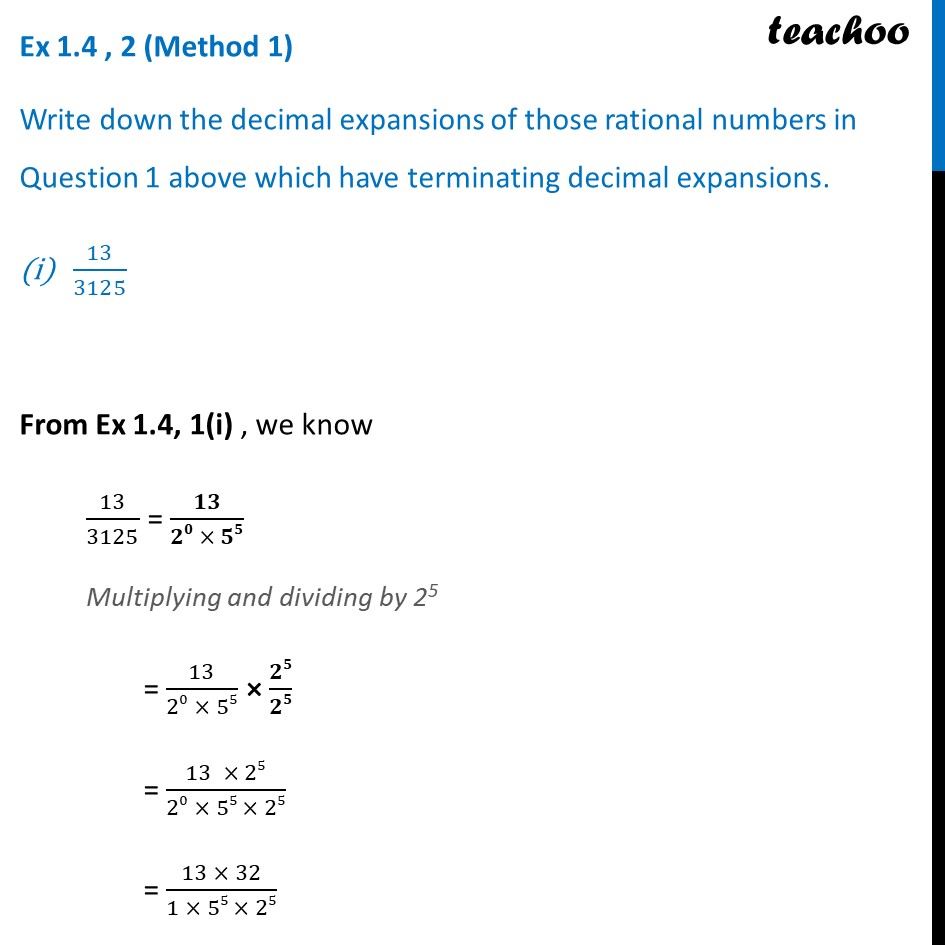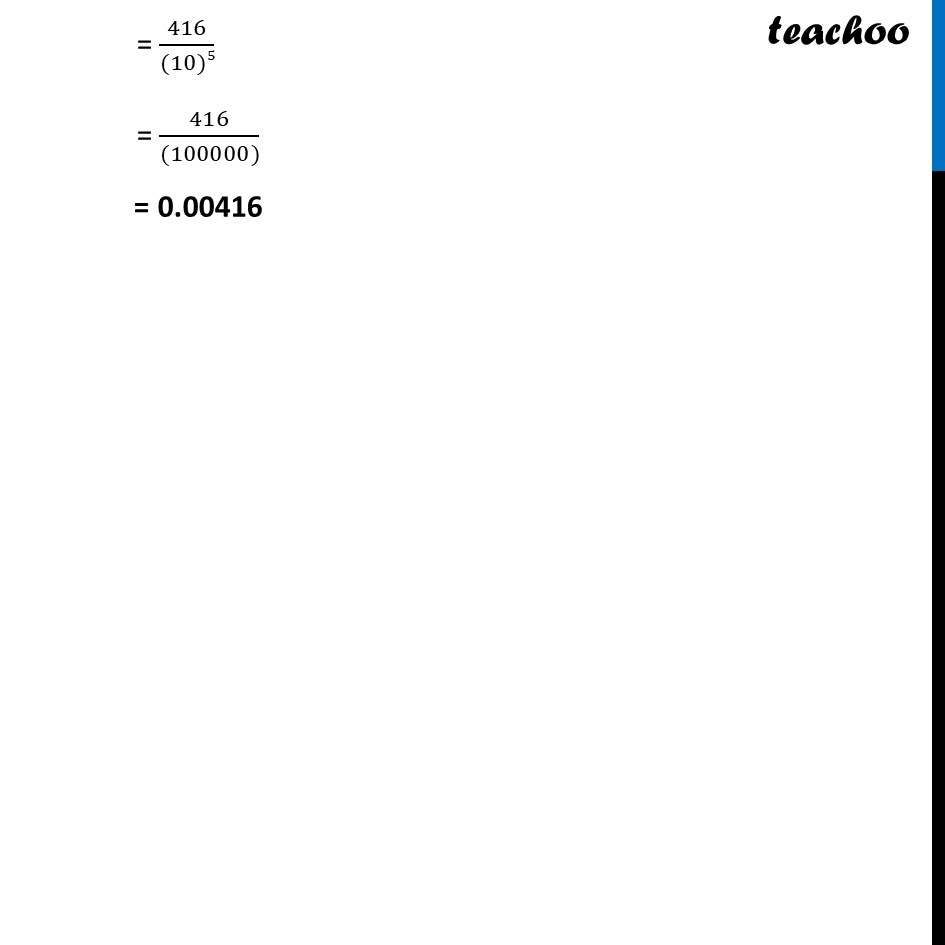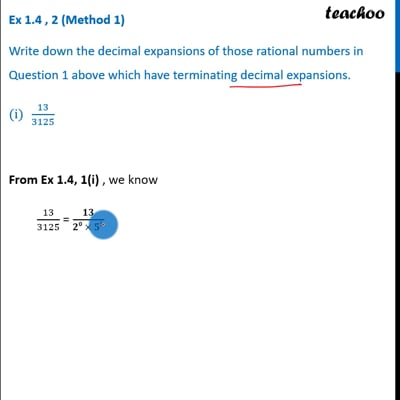This video is only available for Teachoo black users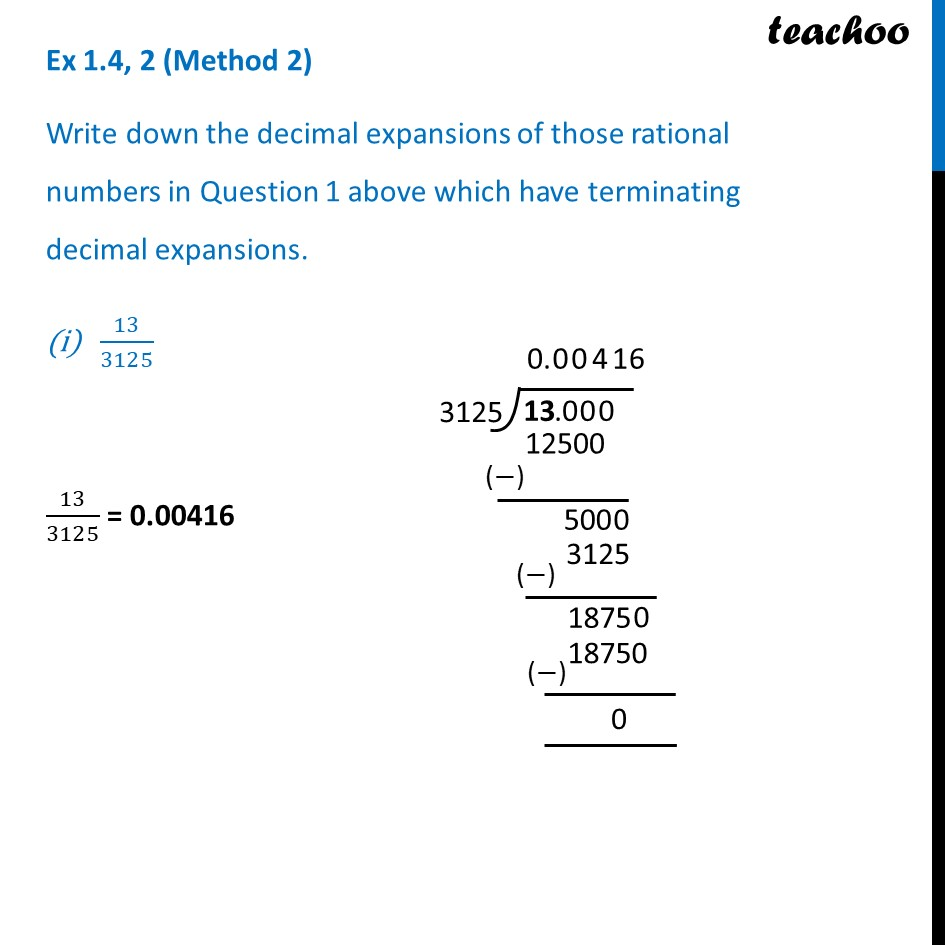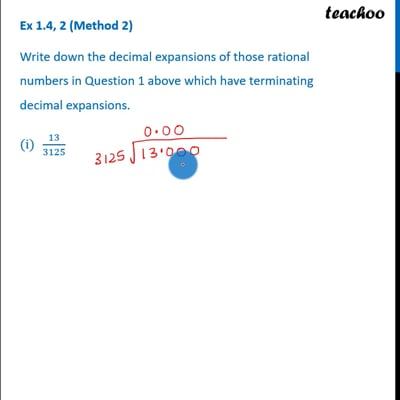This video is only available for Teachoo black users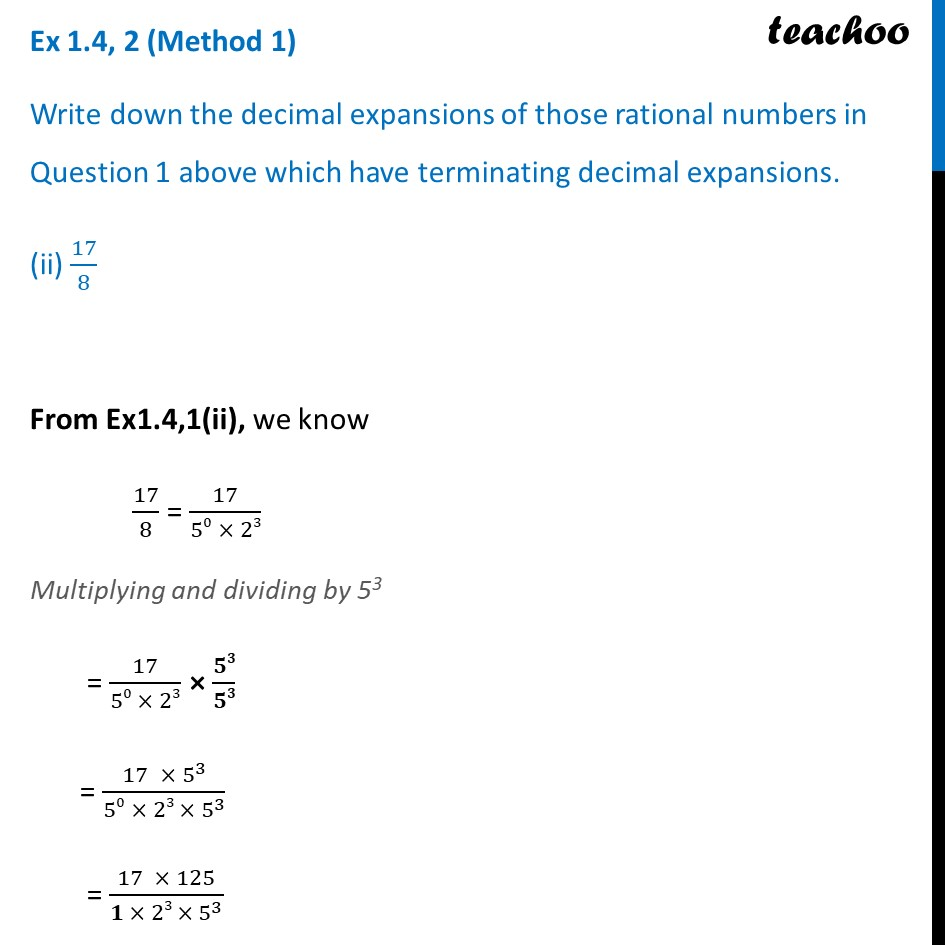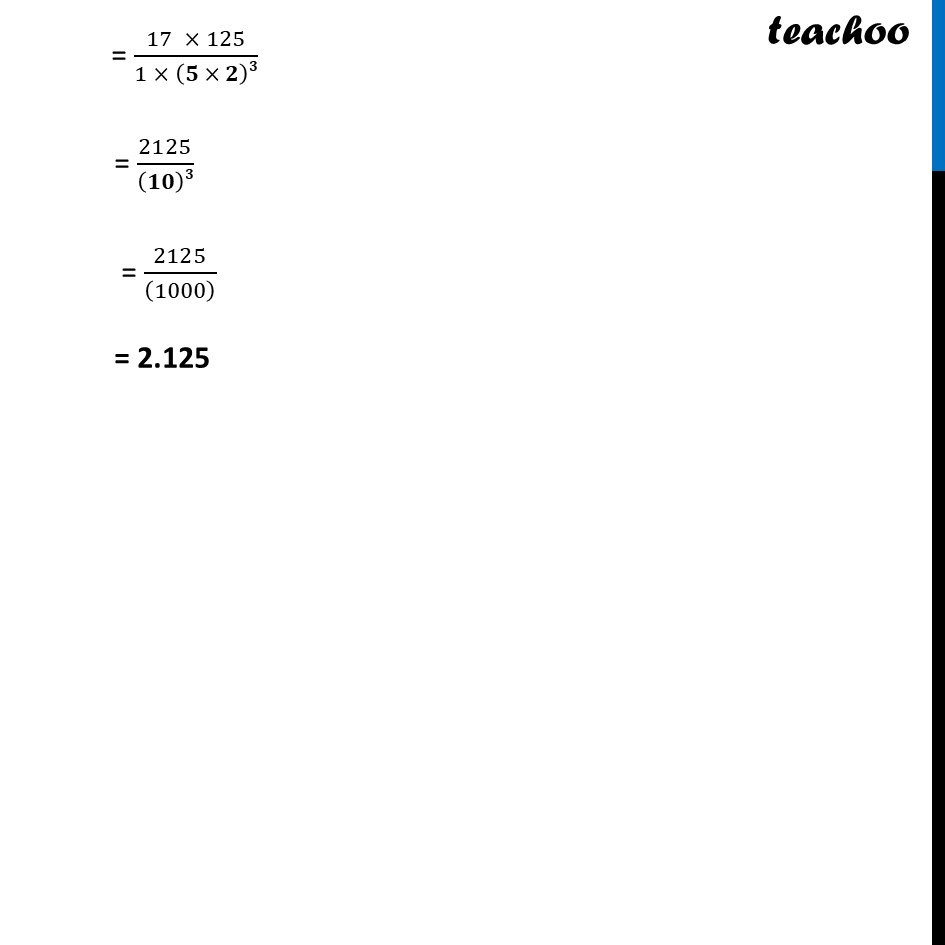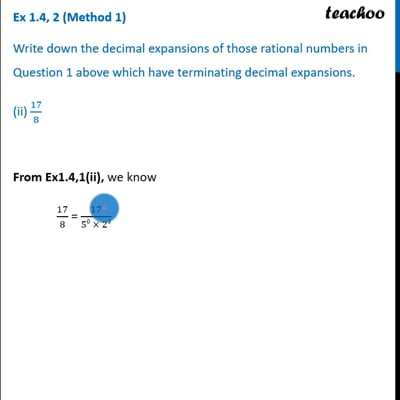This video is only available for Teachoo black users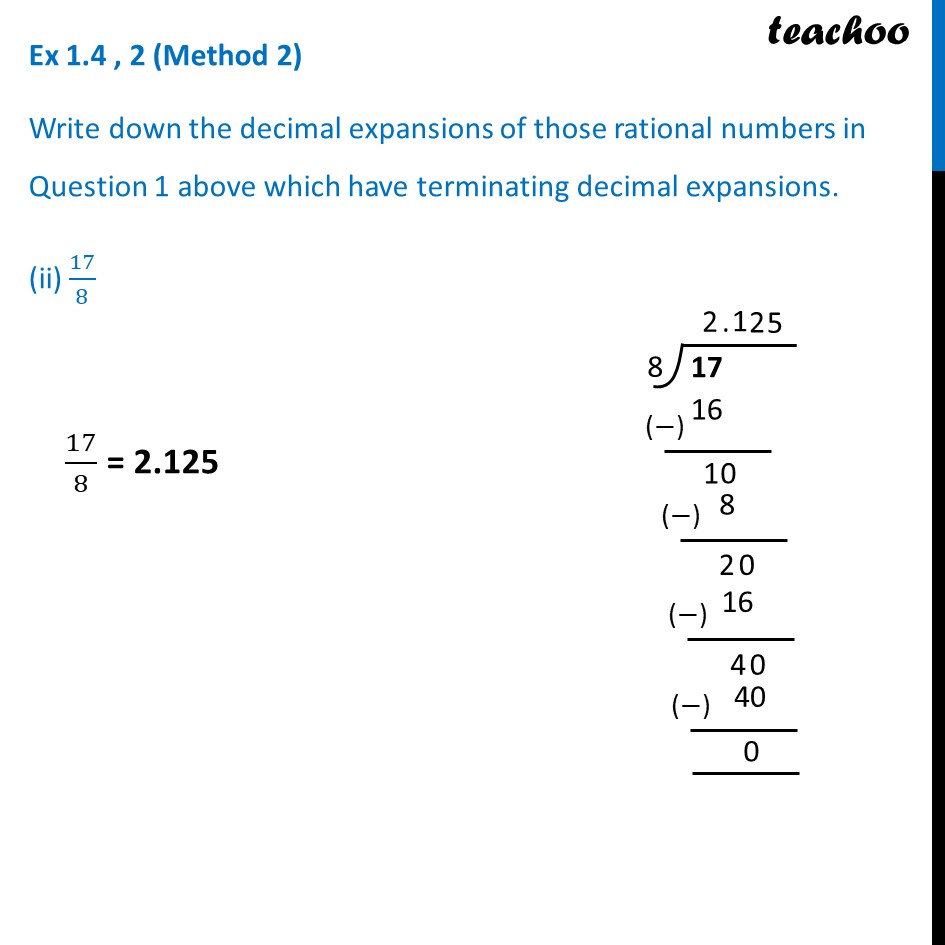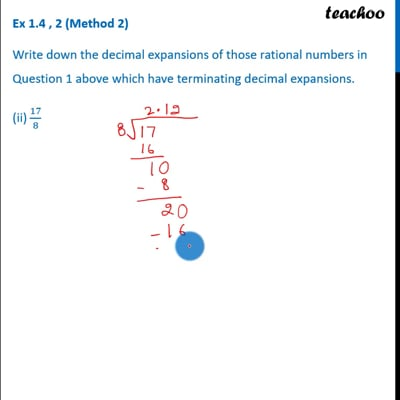This video is only available for Teachoo black users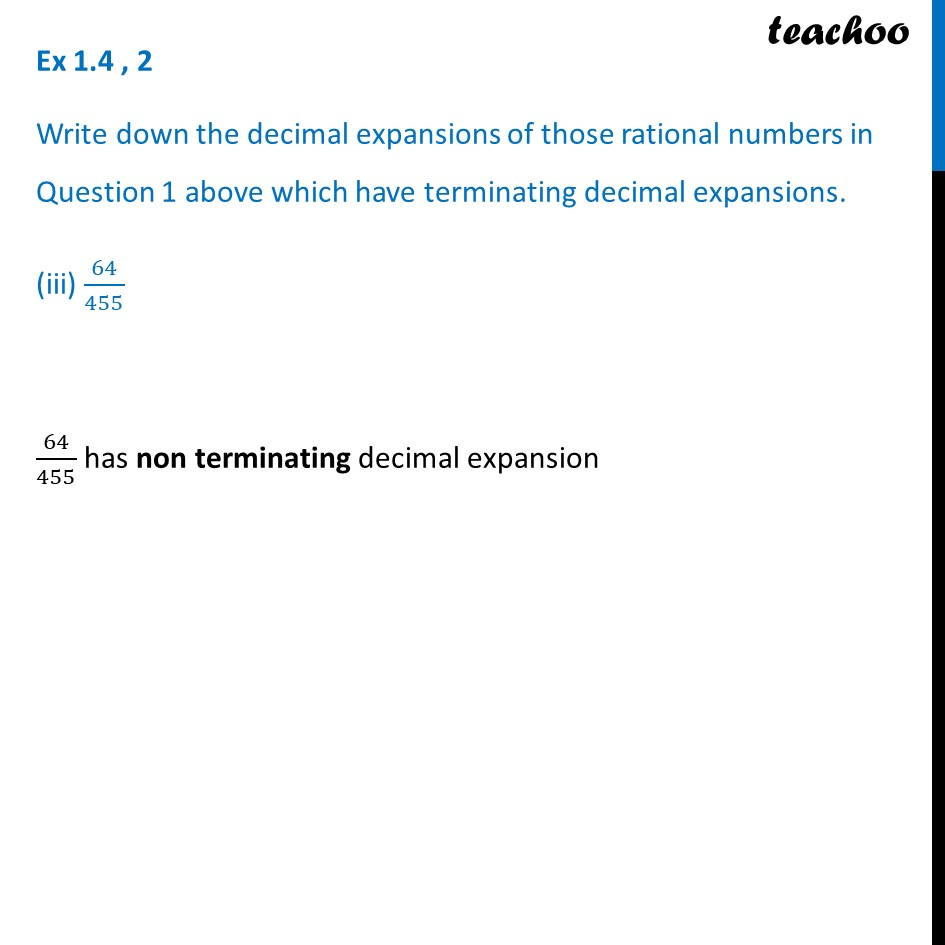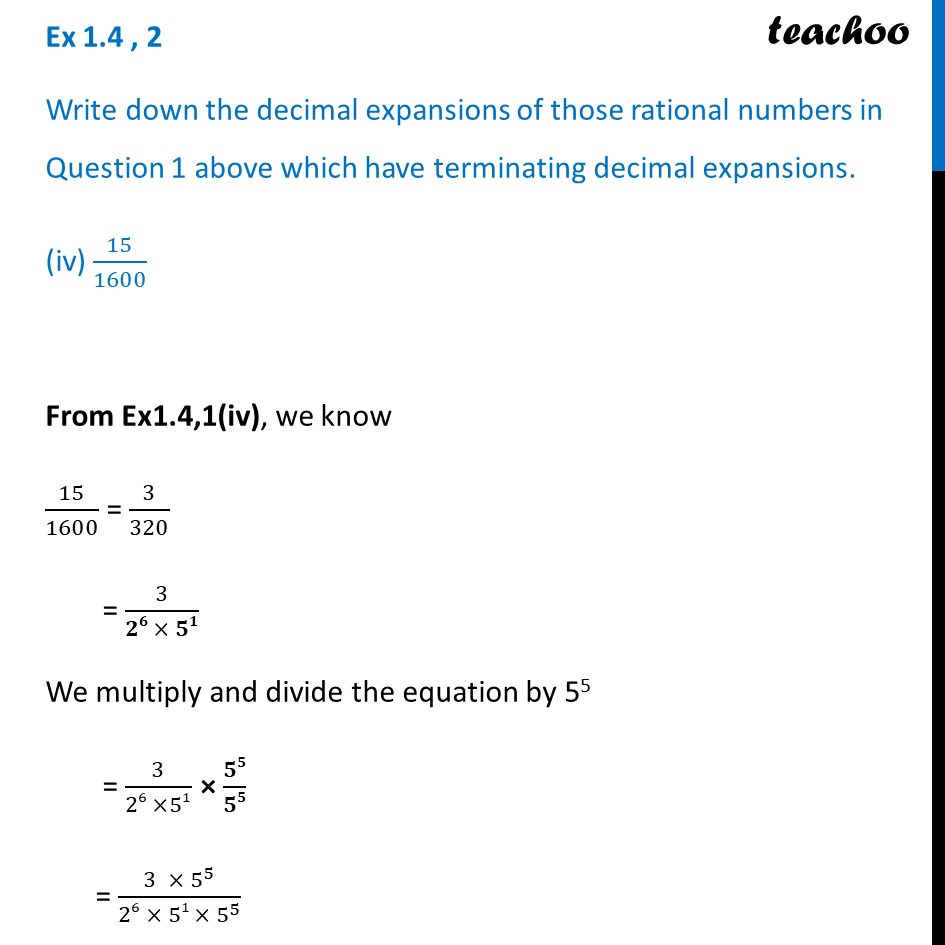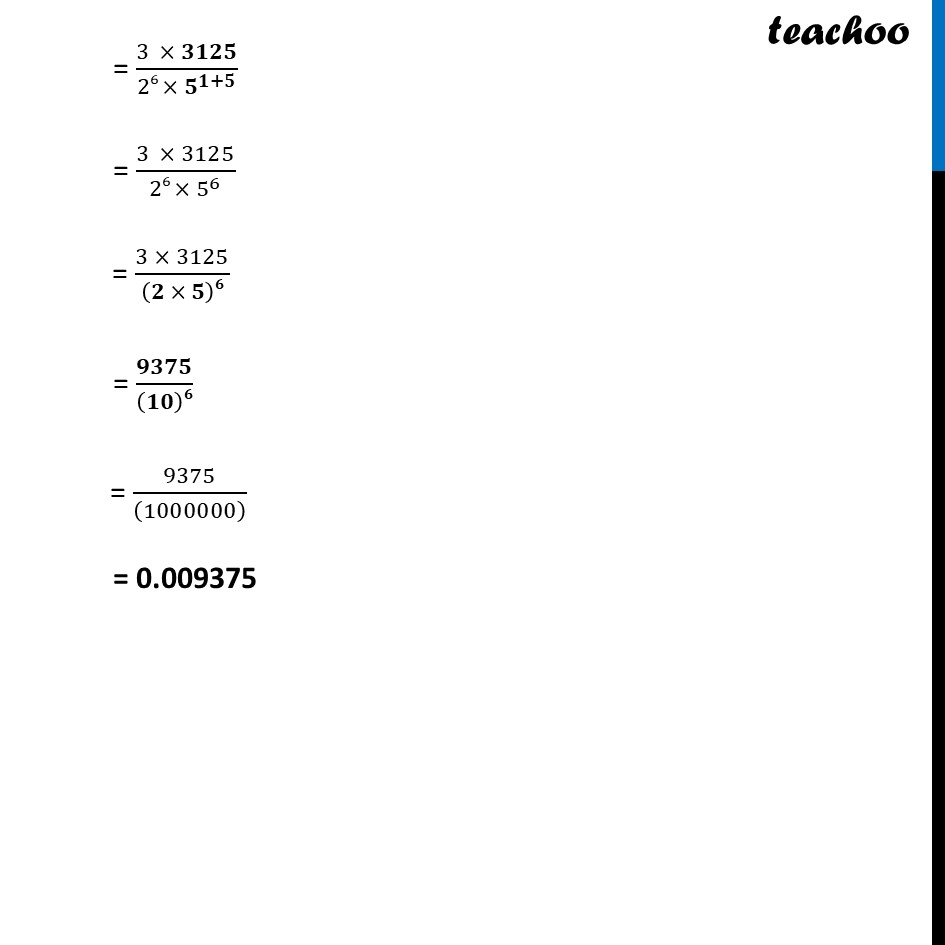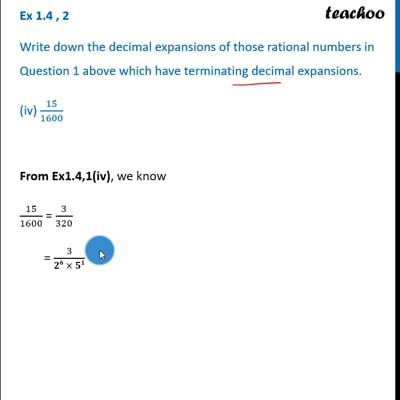This video is only available for Teachoo black users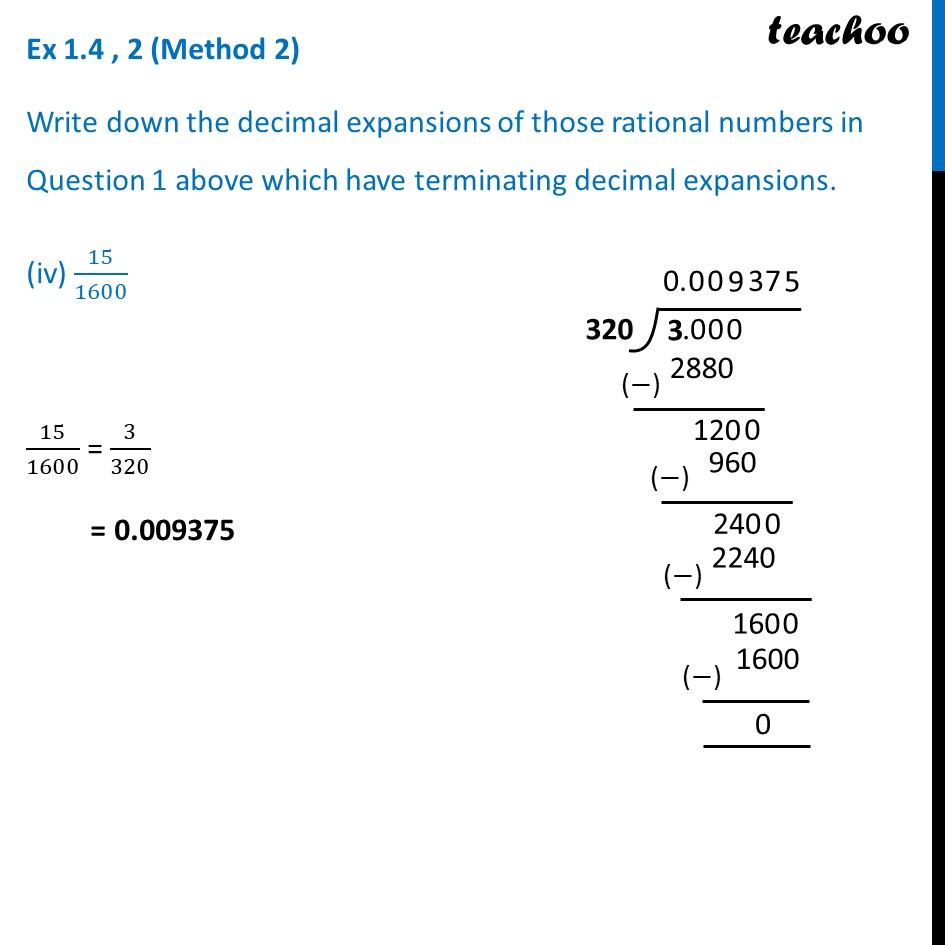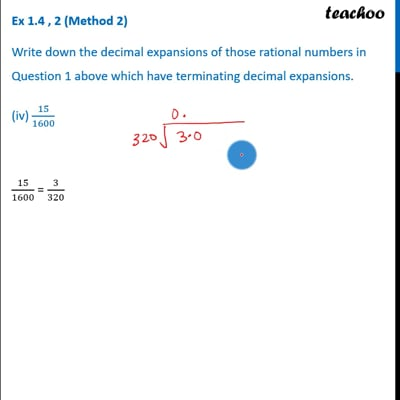This video is only available for Teachoo black users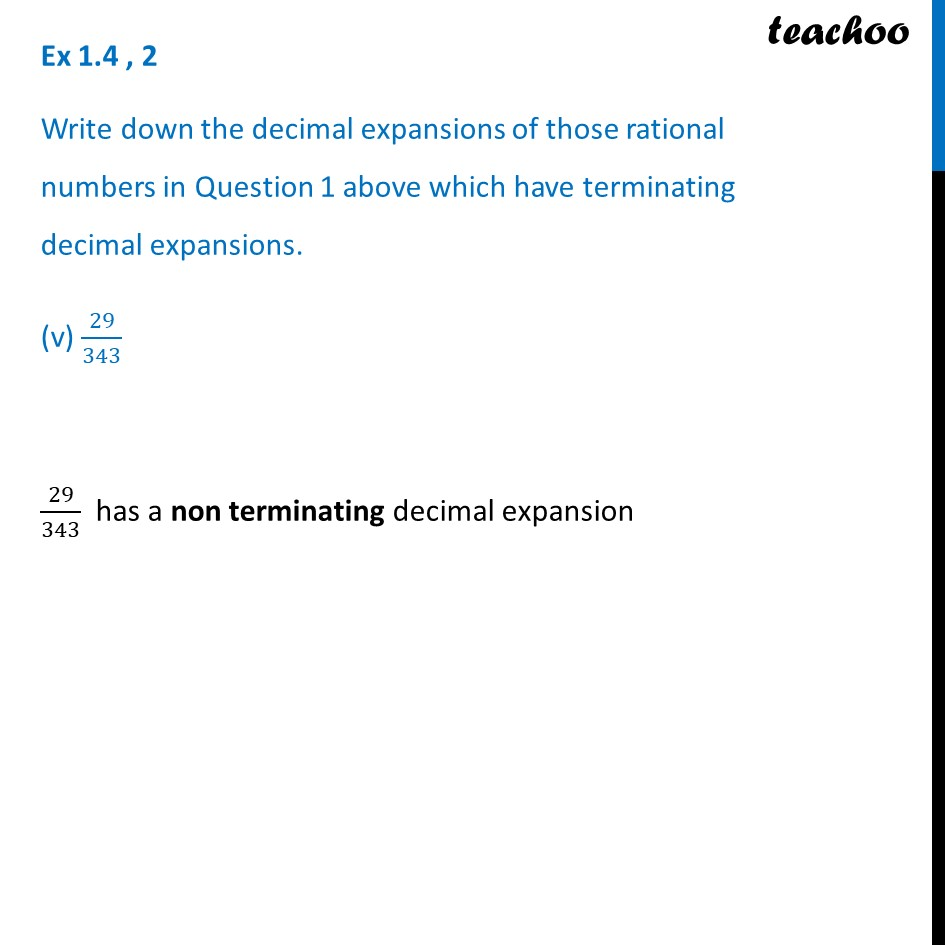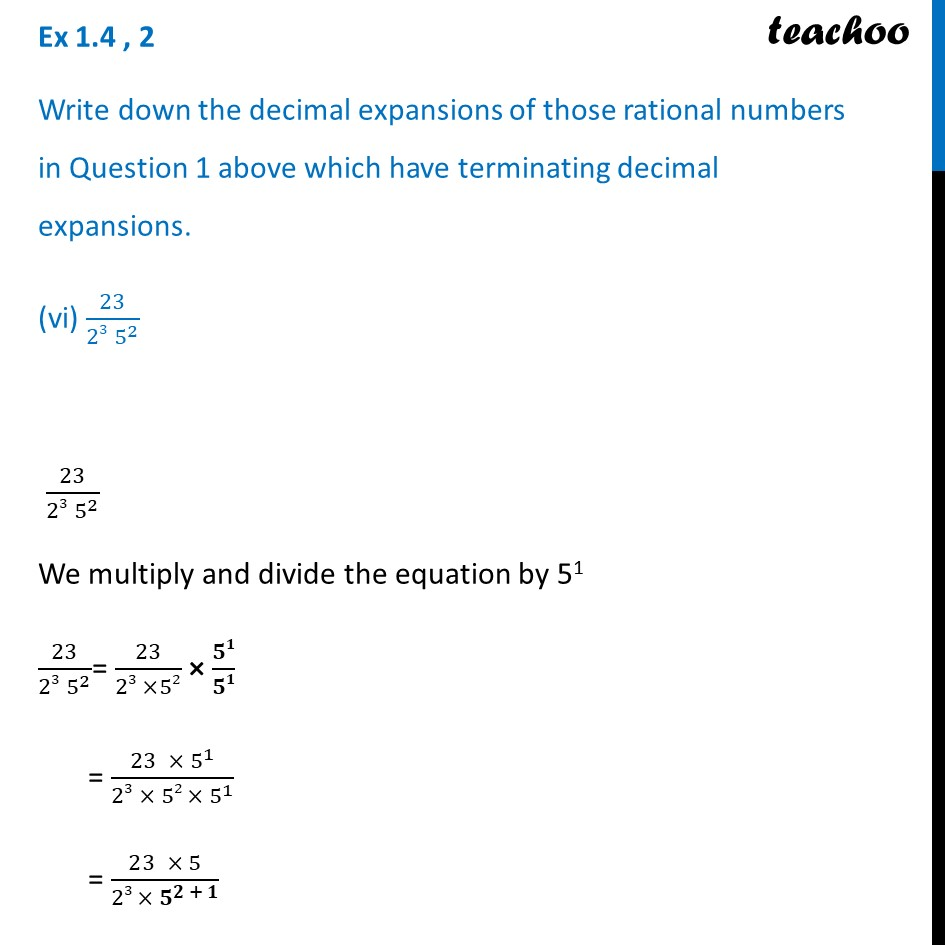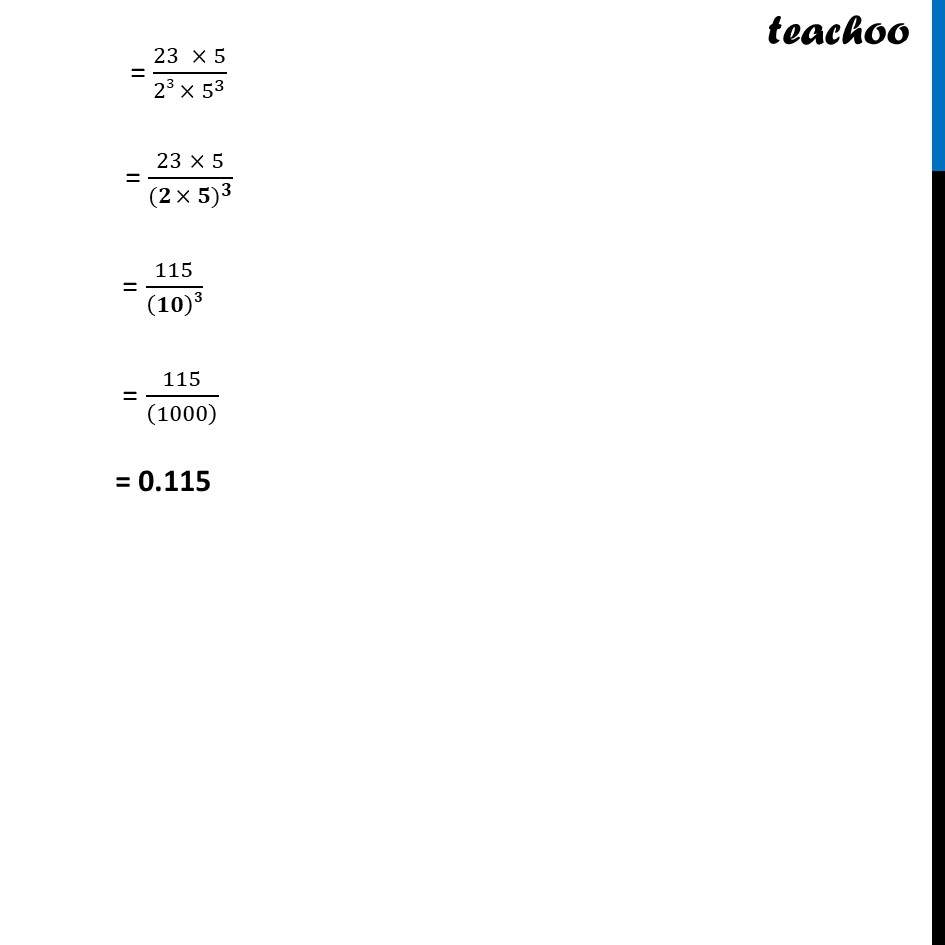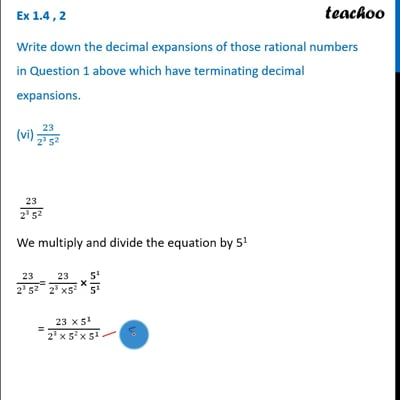This video is only available for Teachoo black users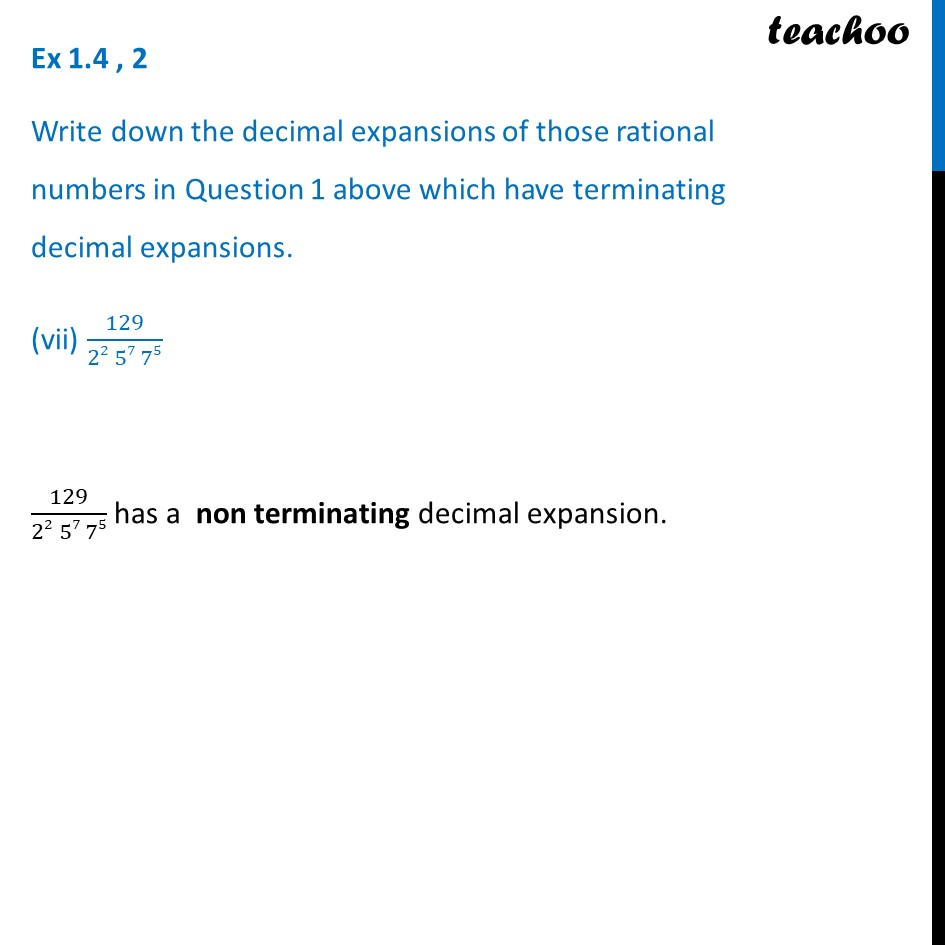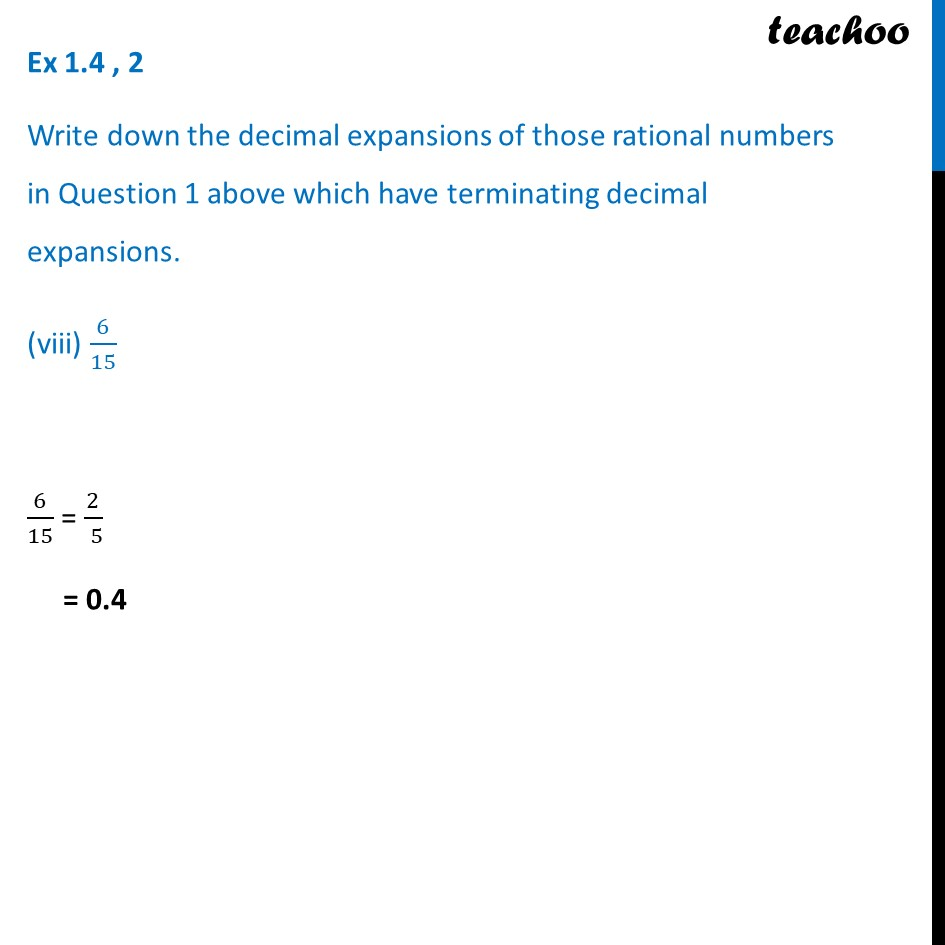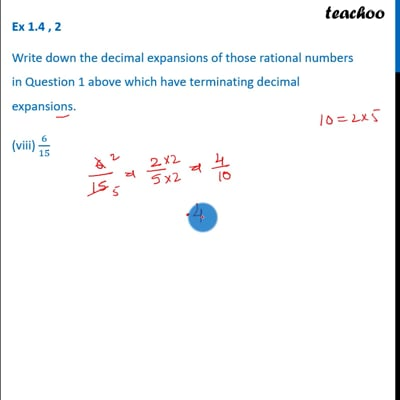This video is only available for Teachoo black users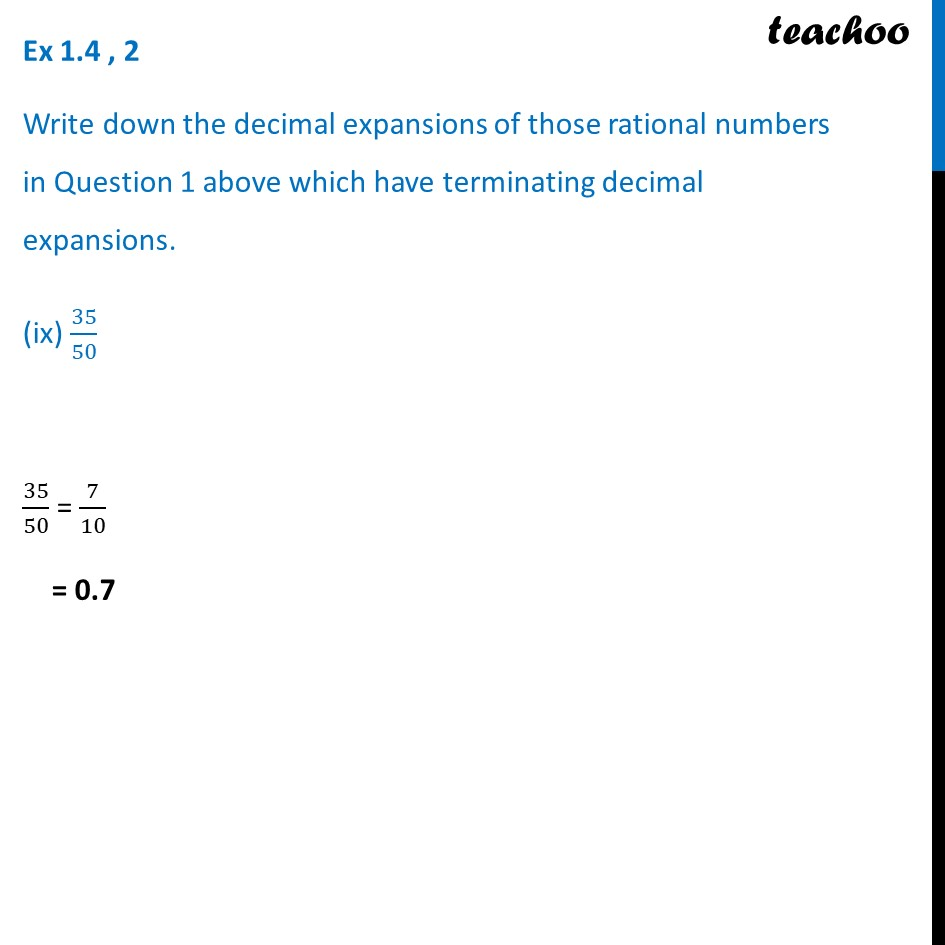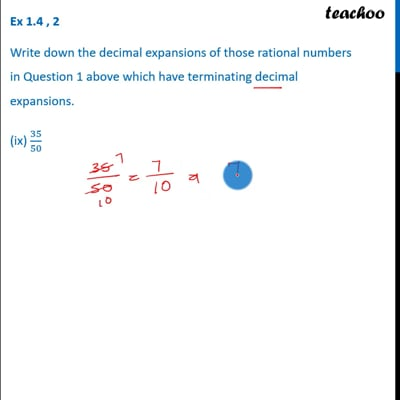This video is only available for Teachoo black users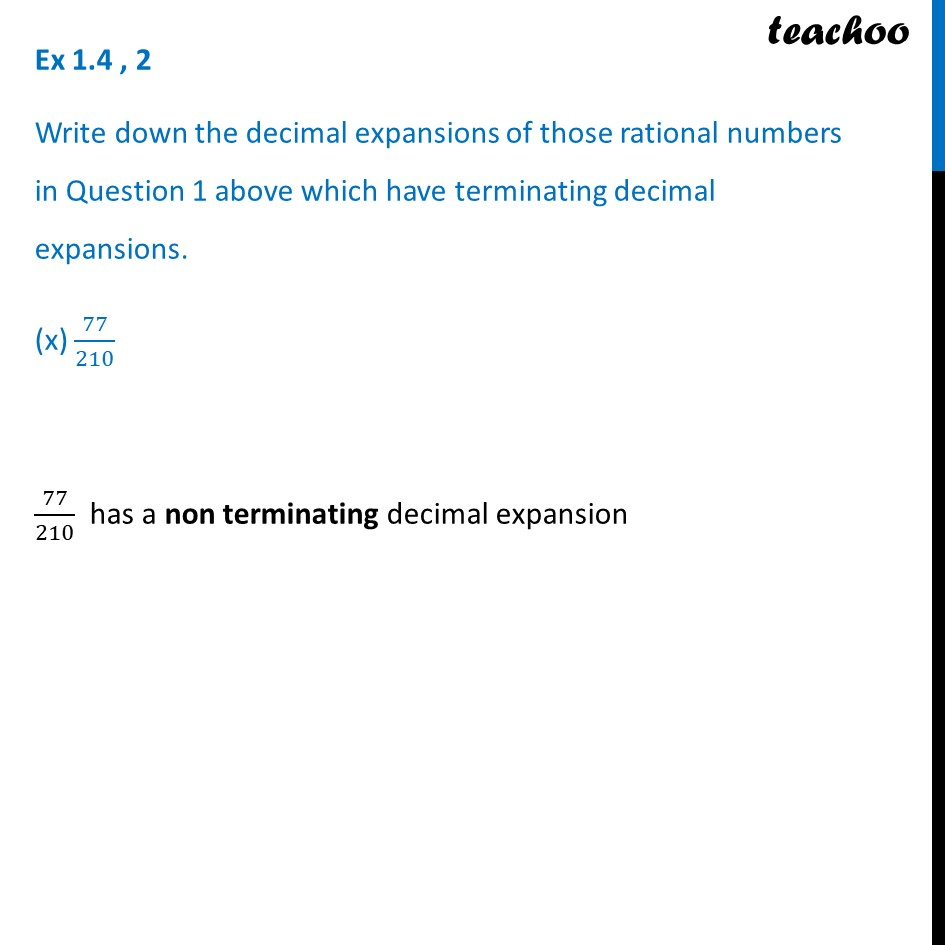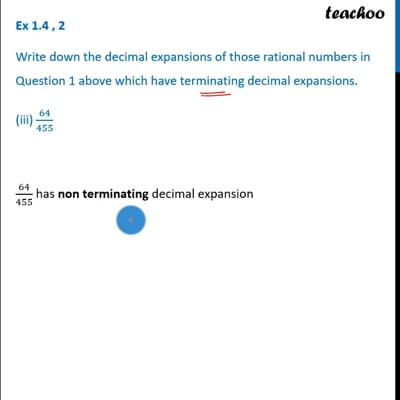This video is only available for Teachoo black users

### Transcript

Ex 1.4 , 2 (Method 1) Write down the decimal expansions of those rational numbers in Question 1 above which have terminating decimal expansions. 13/3125 From Ex 1.4, 1(i) , we know 13/3125 = 𝟏𝟑/(𝟐𝟎 × 𝟓𝟓) Multiplying and dividing by 25 = 13/(20 × 55) × 𝟐𝟓/𝟐𝟓 = (13 × 25)/(20 × 55 × 25) = (13 × 32)/(1 × 55 × 25) = 416/((10)5) = 416/((100000)) = 0.00416 Ex 1.4, 2 (Method 2) Write down the decimal expansions of those rational numbers in Question 1 above which have terminating decimal expansions. 13/3125 13/3125 = 0.00416 Ex 1.4, 2 (Method 1) Write down the decimal expansions of those rational numbers in Question 1 above which have terminating decimal expansions. (ii) 17/8 From Ex1.4,1(ii), we know 17/8 = 17/(50 × 23) Multiplying and dividing by 53 = 17/(50 × 23) × 𝟓𝟑/𝟓𝟑 = (17 × 5^3)/(50 × 23 × 5^3 ) = (17 × 125)/(𝟏 × 23 × 5^3 ) = (17 × 125)/(1 × (𝟓 × 𝟐)𝟑) = 2125/(𝟏𝟎)𝟑 = 2125/((1000) ) = 2.125 Ex 1.4 , 2 (Method 2) Write down the decimal expansions of those rational numbers in Question 1 above which have terminating decimal expansions. (ii) 17/8 17/8 = 2.125 Ex 1.4 , 2 Write down the decimal expansions of those rational numbers in Question 1 above which have terminating decimal expansions. (iii) 64/455 64/455 has non terminating decimal expansion Ex 1.4 , 2 Write down the decimal expansions of those rational numbers in Question 1 above which have terminating decimal expansions. (iv) 15/1600 From Ex1.4,1(iv), we know 15/1600 = 3/320 = 3/(𝟐𝟔 × 𝟓𝟏) We multiply and divide the equation by 55 = 3/(26 ×51) × 𝟓𝟓/𝟓𝟓 = (3 × 5^5)/(26 × 51 × 5^5 ) = (3 × 𝟑𝟏𝟐𝟓)/(26 × 𝟓^(𝟏+𝟓) ) = (3 × 3125)/(26 〖× 5〗^6 ) = (3 × 3125)/(𝟐 × 𝟓)𝟔 = 𝟗𝟑𝟕𝟓/(𝟏𝟎)𝟔 = 9375/((1000000) ) = 0.009375 Ex 1.4 , 2 (Method 2) Write down the decimal expansions of those rational numbers in Question 1 above which have terminating decimal expansions. (iv) 15/1600 15/1600 = 3/320 = 0.009375 Ex 1.4 , 2 Write down the decimal expansions of those rational numbers in Question 1 above which have terminating decimal expansions. (v) 29/343 29/343 has a non terminating decimal expansion Ex 1.4 , 2 Write down the decimal expansions of those rational numbers in Question 1 above which have terminating decimal expansions. (vi) 23/(23 5^2 ) 23/(23 5^2 ) We multiply and divide the equation by 51 23/(23 5^2 )= 23/(23 ×52) × 𝟓𝟏/𝟓𝟏 = (23 × 5^1)/(23 × 52 〖× 5〗^1 ) = (23 × 5)/(23 〖× 𝟓〗^(𝟐 + 𝟏) ) = (23 × 5)/(23 〖× 5〗^3 ) = (23 × 5)/((𝟐 × 〖𝟓)〗^𝟑 ) = 115/(𝟏𝟎)𝟑 = 115/((1000) ) = 0.115 Ex 1.4 , 2 Write down the decimal expansions of those rational numbers in Question 1 above which have terminating decimal expansions. (vii) 129/(22 57 75) 129/(22 57 75) has a non terminating decimal expansion. Ex 1.4 , 2 Write down the decimal expansions of those rational numbers in Question 1 above which have terminating decimal expansions. (viii) 6/15 6/15 = 2/( 5) = 0.4 Ex 1.4 , 2 Write down the decimal expansions of those rational numbers in Question 1 above which have terminating decimal expansions. (ix) 35/50 35/50 = 7/10 = 0.7 Ex 1.4 , 2 Write down the decimal expansions of those rational numbers in Question 1 above which have terminating decimal expansions. (x) 77/210 77/210 has a non terminating decimal expansion